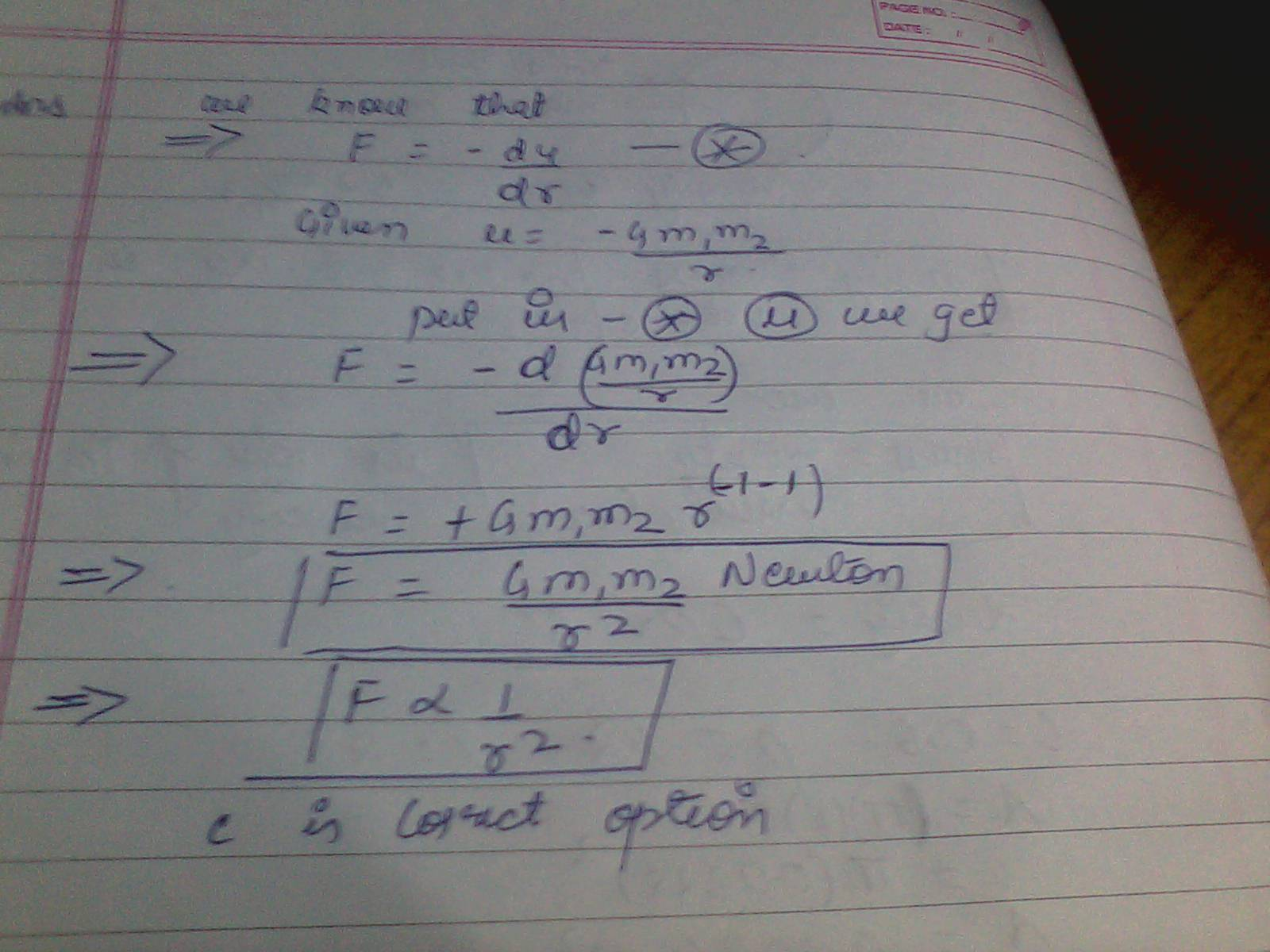# The gravitational force between two objects of masses m1 and m2 that are separated by distance r is

The gravitational force between two objects of masses

m1

and
m2

that are separated by distance r
is?
The gravitational force between two objects of masses
m1
m 1
and
m2
m 2
that are separated by distance r
is?

a: proportion to r
a: proportion to r
b:proportional 1/r
b:proportional 1/r
c:proportional 1/r^2
c:proportional 1/r^2
d:(m1+m2)g
d:(m1+m2)g
d:(m1+m2)G
d:(m1+m2)G

equation is U=-Gm1*m2/r
equation is U=-Gm1*m2/r

Problem is is that I want to choose B as my answer but A also
sounds correct. Which one is right?

Problem is is that I want to choose B as my answer but A also
sounds correct. Which one is right?

Answer is C ….
equation is U=Gm1m2/r^2 hence it is proportional to 1/r^2. Hence
option:c